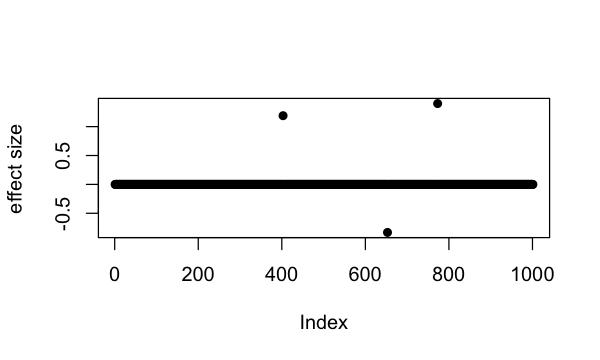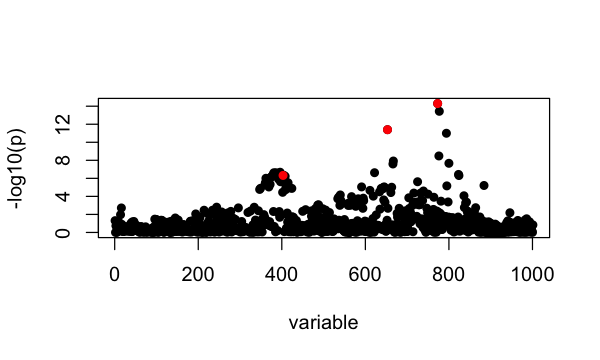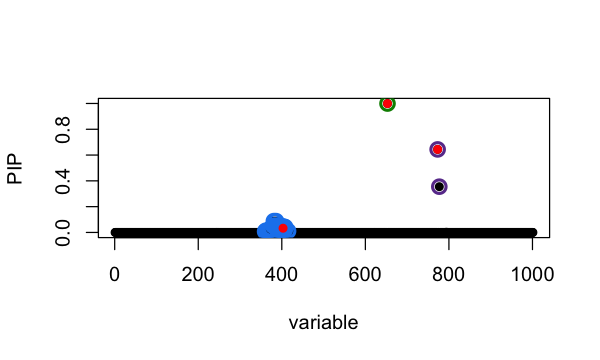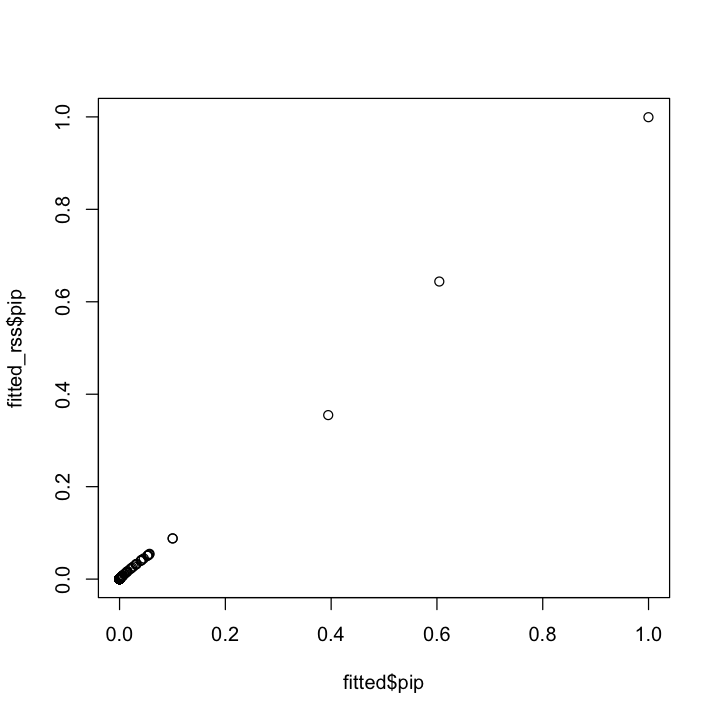# Fine-mapping with summary statistics

#### 2021-06-07

This vignette demonstrates how to use susieR with “summary statistics” in the context of genetic fine-mapping. We use the same simulated data as in fine mapping vignette. The simulated data is expression level of a gene ($$y$$) in $$N \approx 600$$ individuals. We want to identify with the genotype matrix $$X_{N\times P}$$ ($$P=1001$$) the genetic variables that causes changes in expression level. The data-set is shipped with susieR. It is simulated to have exactly 3 non-zero effects.

library(susieR)
set.seed(1)

## The data-set

data(N3finemapping)
attach(N3finemapping)

Notice that we’ve simulated 2 sets of $$Y$$ as 2 simulation replicates. Here we’ll focus on the first data-set.

dim(Y)
#  574   2

Here are the 3 “true” signals in the first data-set:

b <- true_coef[,1]
plot(b, pch=16, ylab='effect size')which(b != 0)
#  403 653 773

So the underlying causal variables are 403, 653 and 773.

## Summary statistics from simple regression

Summary statistics of genetic association studies typically contain effect size ($$\hat{\beta}$$ coefficient from regression), p-value and minor allele frequencies. These information can be used to perform fine-mapping with given an additional input of correlation matrix between variables. The correlation matrix in genetics is typically referred to as LD matrix (LD for linkage disequilibrium). One may use external reference panels to estimate it when this matrix cannot be obtained from samples directly. Caution that LD matrix here has to be correlation matrix $$r$$, not $$r^2$$ or $$abs(r)$$.

univariate_regression function can be used to compute summary statistics by fitting univariate simple regression variable by variable. The results are $$\hat{\beta}$$ and $$SE(\hat{\beta})$$ from which z-scores can be derived. Alternatively you can obtain z-scores from $$\hat{\beta}$$ and p-values if you are provided with those information. Again we focus only on results from the first data-set:

sumstats <- univariate_regression(X, Y[,1])
z_scores <- sumstats$betahat / sumstats$sebetahat
susie_plot(z_scores, y = "z", b=b)For this example the correlation matrix can be computed directly from data provide,

R <- cor(X)

## Fine-mapping with susieR using summary statistics

For starters, we assume there are at most 10 causal variables, i.e. set L = 10, although SuSiE is generally robust to the choice of L.

SuSiE regression with summary statistics is implemented as susie_rss function,

fitted_rss <- susie_rss(z_scores, R, L = 10)

Using summary function, we can examine the posterior inclusion probability (PIP) for each variable, and the 95% credible sets.

Here, we are the 95% credible sets.

summary(fitted_rss)$cs # cs cs_log10bf cs_avg_r2 cs_min_r2 # 1 2 3.113066 1.0000000 1.0000000 # 2 1 5.784413 0.9816575 0.9634847 # 3 3 2.590789 0.9188853 0.6907024 # variable # 1 653 # 2 773,777 # 3 360,362,365,368,372,373,374,379,381,383,384,386,387,388,389,391,392,396,397,398,399,400,401,403,404,405,407,408,415 The 3 causal signals have been captured by the 3 CS reported here. The 3rd CS contains many variables, including the true causal variable 403. We can also plot the posterior inclusion probability (PIP), susie_plot(fitted_rss, y="PIP", b=b)The true causal variables are colored red. The 95% CS identified are circled in different colors. The result should be very similar to using the individual level data for this example, as the z-scores and correlation matrix (LD reference) come from the same genotype data-set. fitted = susie(X, Y[,1], L = 10, estimate_residual_variance = TRUE, estimate_prior_variance = TRUE) plot(fitted$pip, fitted_rss\$pip, ylim=c(0,1))## Session information

Here are some details about the computing environment, including the versions of R, and the R packages, used to generate these results.

sessionInfo()
# R version 3.6.2 (2019-12-12)
# Platform: x86_64-apple-darwin15.6.0 (64-bit)
# Running under: macOS Catalina 10.15.7
#
# Matrix products: default
# BLAS:   /Library/Frameworks/R.framework/Versions/3.6/Resources/lib/libRblas.0.dylib
# LAPACK: /Library/Frameworks/R.framework/Versions/3.6/Resources/lib/libRlapack.dylib
#
# locale:
#  en_US.UTF-8/en_US.UTF-8/en_US.UTF-8/C/en_US.UTF-8/en_US.UTF-8
#
# attached base packages:
#  stats     graphics  grDevices utils     datasets  methods   base
#
# other attached packages:
#  susieR_0.11.42
#
# loaded via a namespace (and not attached):
#   Rcpp_1.0.5       knitr_1.26       magrittr_1.5     tidyselect_0.2.5
#   munsell_0.5.0    colorspace_1.4-1 lattice_0.20-38  R6_2.4.1
#   rlang_0.4.5      highr_0.8        plyr_1.8.5       dplyr_0.8.3
#  stringr_1.4.0    tools_3.6.2      grid_3.6.2       gtable_0.3.0
#  xfun_0.11        irlba_2.3.3      htmltools_0.4.0  assertthat_0.2.1
#  yaml_2.2.0       digest_0.6.23    tibble_2.1.3     lifecycle_0.1.0
#  crayon_1.3.4     mixsqp_0.3-46    Matrix_1.2-18    purrr_0.3.3
#  ggplot2_3.3.0    glue_1.3.1       evaluate_0.14    rmarkdown_2.3
#  stringi_1.4.3    compiler_3.6.2   pillar_1.4.3     scales_1.1.0
#  reshape_0.8.8    pkgconfig_2.0.3